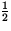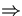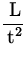# Dimensional Analysis

Definition: The Dimension is the qualitative nature of a physical quantity (length, mass, time).

Square brackets denote the dimension or units of a physical quantity:

 quantity dimension SI units area [A] = L 2 m 2 volume [V]=L 3 m 3 velocity [v] = L/t m/s acceleration [a] = L/t 2 m/s 2 mass [m] = M kg

Idea: Dimensional analysis can be used to derive or check formulas by treating dimensions as algebraic quantities. Quantities can be added or subtracted only if they have the same dimensions, and quantities on two sides of an equation must have the same dimensions.

Note: Dimensional analysis can't give numerical factors. For Example: The distance (x) travelled by a car in a given time (t) , starting from rest and moving with constant acceleration (a) is given by, x =at 2. We can check this equation with dimensional analysis:

 l.h.s.[x] = L r.h.s.=[a][t 2] =t 2 = L.
Since the dimension of the left hand side (l.h.s.) of the equation is the same as that on the right hand side (r.h.s.), the equation is said to be dimensionally consistent.Next: Conversion of Units Up: Introduction Previous: Scientific Notation